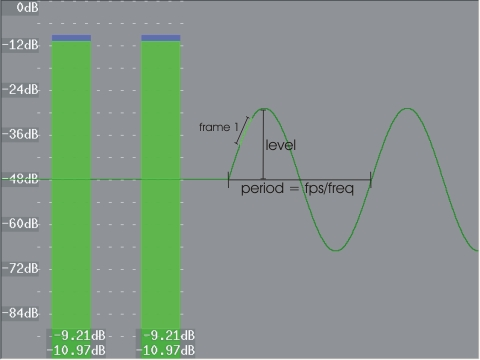# Tone

AviSynth+

This will generate sound (a waveform) at a given frequency for a given length of time in seconds.

Tone( [ float length, float frequency, int samplerate, int channels, string type, float level ] )

float  length = 10.0
Length of time in seconds.
float  frequency = 440
Tone frequency.
int  samplerate = 48000
Audio sample rate.
int  channels = 2
Number of audio channels.
string  type = "Sine"
"Silence", "Sine", "Noise", "Square", "Triangle" or "Sawtooth".
float  level = 1.0
The amplitude of the waveform. Default = 1.0 (or 0dB, the maximum level possible without clipping).
For a particular decibel level, use the equation level = 10 dB / 20
For example, create a -12dB tone with level = 10-12/20 or 0.251.

The audio sample type is 32-bit float. Use ConvertAudio if this is not what you want.

#### DiscussionTone(frequency=2, samplerate=48000, channels=2, type="sine", level=0.4)
In the figure above, a sinus is generated (on a gray clip with framerate 24 fps). The period of the waveform (in frames) is the framerate divided by frequency (or fps/freq, which is 24/2=12 frames in our example). The part of the graph which is light-green represents all samples of the frame under consideration (which is frame 1 here). The number of samples in a particular frame is given by the samplerate divided by the framerate (which is 48000/24 = 2000 samples in our example).
More generally, the waveform above is described by
g(n,s) = level · sin(2 · pi · (frequency · n / framerate + s · frequency / samplerate))
with n the frame and s the sample under consideration (note that s runs from 0 to samplerate/framerate - 1).
In the example above, this reduces to
g(n,s) = 0.4 · sin(2 · pi · (2 · n / 24 + s · 2 / 48000))
with n the frame and s the sample under consideration (note that s runs from 0 to 1999).
Note that the bars are made with Histogram and the graph with the AudioGraph plugin.

#### Examples

We Wish You a Merry Christmas, in harmony (author hanzfrunz)

#### Changes

 v2.56 Added Level. v2.54 Initial Release.